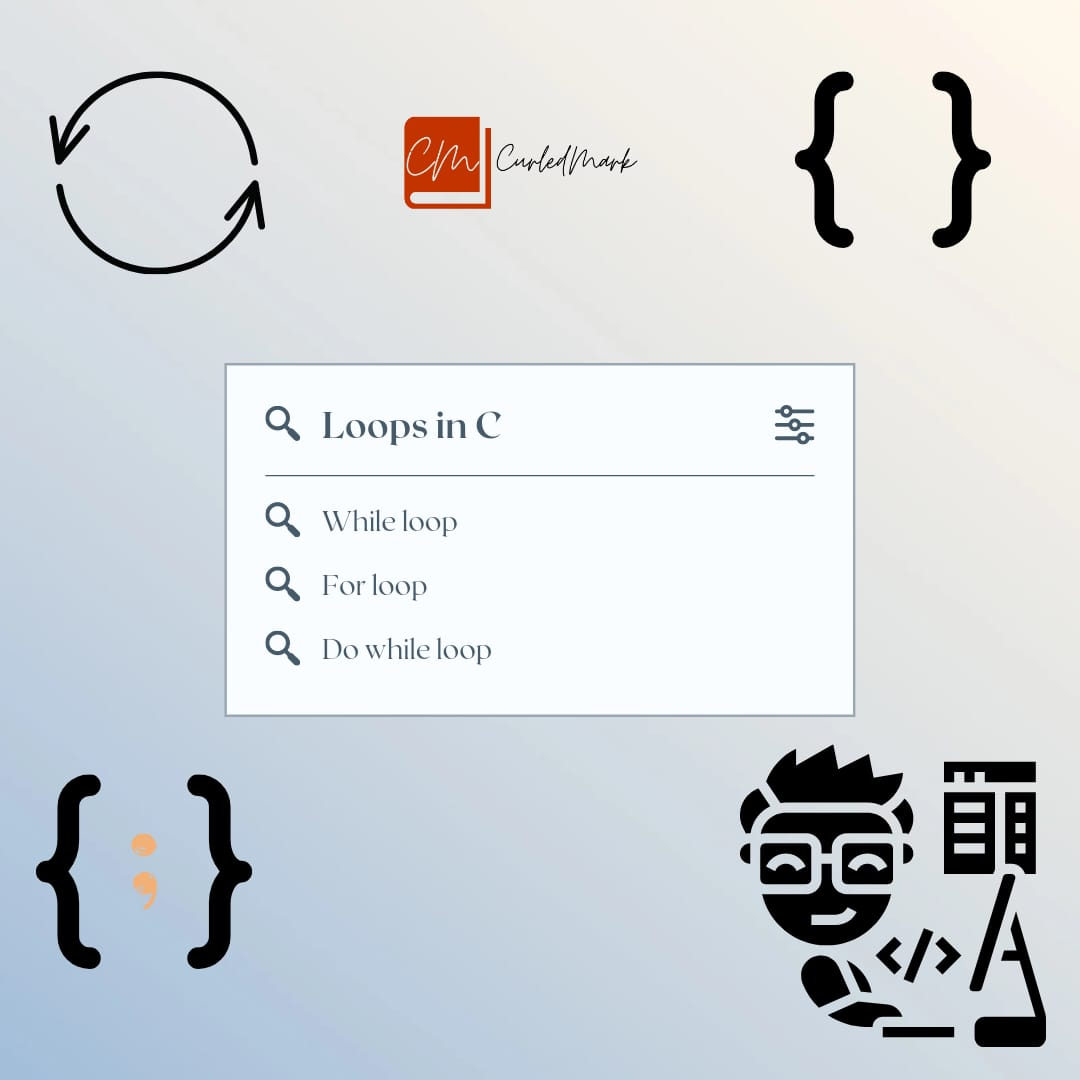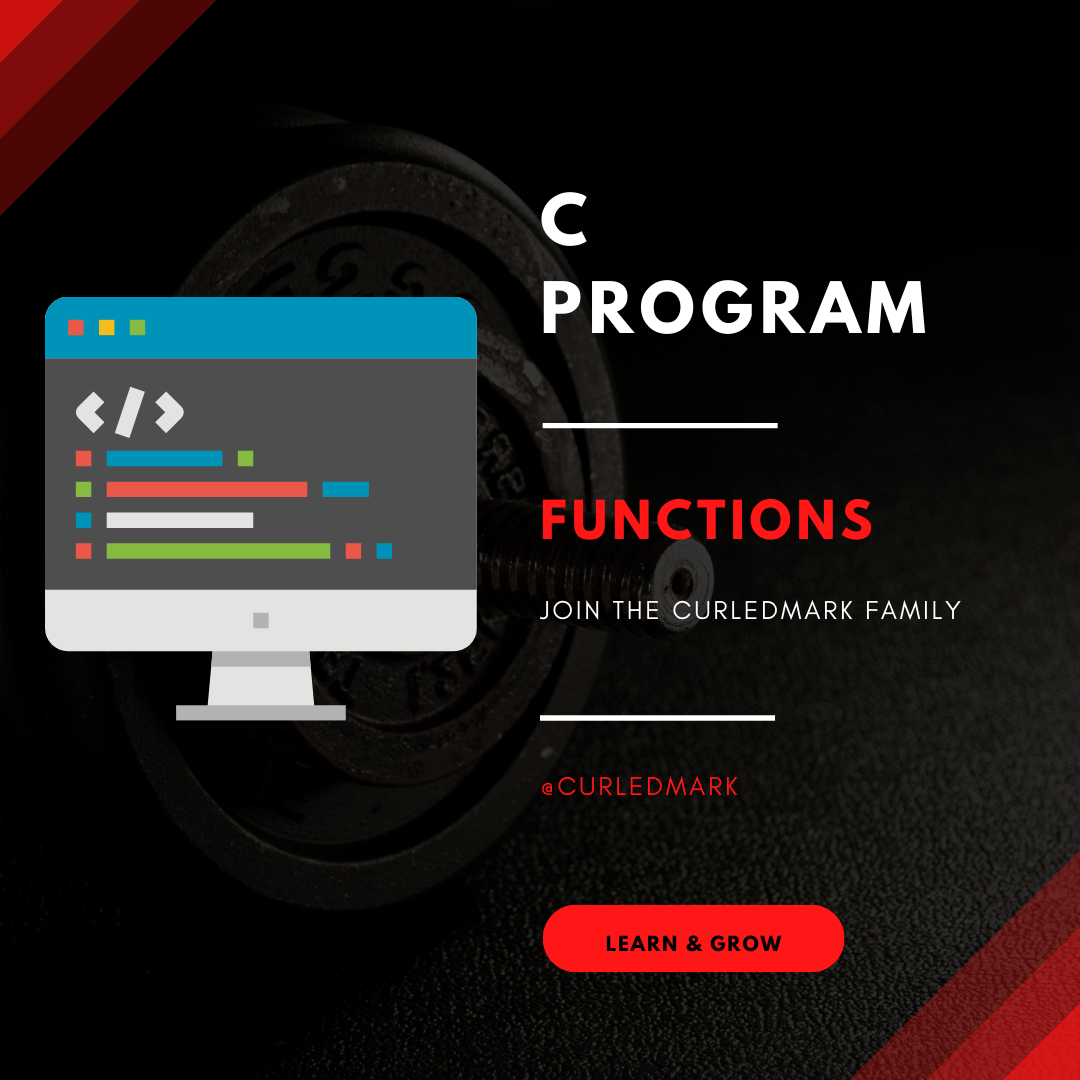September 25, 2022# Prime, reverse,power Programs in C Programming

Prime Number

Prime Number is a number that is divisible by 1 or itself. Negative numbers, zero, and one are not prime numbers.

Program 1: Write a program that prints a number to be a prime number or not.

`#include<stdio.h>`

`int main()`

`{`

`int n,y,z,ep;`

`Printf("Enter a number:");`

`Scanf("%d",&n); `

`if(n<=1)`

`{`

`Printf(%d is neither prime number or composite number",n);`

`return 0;`

`}`

`ep=n/2;`

`z=0;`

`y=2;`

`While(y<=ep)`

`{`

`if(n%y == 0)`

`{`

`z=1;`

`break;`

`}`

`y++;`

`}`

`if(z==0)`

`{`

`Printf("%d is a prime number",n); `

`}`

`else`

`{`

`Printf("%d is not a prime number",n);`

`}`

`return 0;`

`}`

Program 2: Write a prime number program that prints all the prime numbers starting from 5 to 20.

`#include<stdio.h>`

`int main()`

`{`

`int n,y,z,ep;`

`Printf("Enter a number:");`

`Scanf("%d",&n); `

`if(n<=1)`

`{`

`Printf(%d is neither prime number or composite number",n);`

`return 0;`

`}`

if(s<=1)

{

n=2;

}

else

{

n=s;

}

While(n<=e)

{

`ep=n/2;`

`z=0;`

`y=2;`

`While(y<=ep)`

`{`

`if(n%y == 0)`

`{`

`z=1;`

`break;`

`}`

`y++;`

`}`

`if(z==0)`

`{`

`Printf("%d\n",n); `

`}`

n++;

`}`

`return 0;`

`}`

Program 3: Write a program to accept a number and count digits.

`#include<stdio.h>`

`int main()`

`{`

`int x, num, dc;`

`printf("Enter a number:");`

`Scanf("%d",&x);`

`if(x<0)`

`{`

`num=x*(-1);`

`}`

`else`

`{`

`num=x;`

`}`

`dc=1;`

`While(num>9)`

`{`

`dc++;`

`num=num/10;`

`}`

`Printf("Number of digits in %d are %d",x,dc);`

`return 0;`

`}`

Program 4: Write a program to reverse a number

` #include<stdio.h>`

`int main()`

`{`

`int x,num,j;`

`Printf("Enter the number to reverse:");`

`Scanf("%d",&x); `

`if(x<0)`

`{`

`j=x(-1);`

`}`

`else`

`{`

`j=x;`

`}`

`num=0;`

`While(j>0)`

`{`

`num=(num*10)+(j%10);`

`j=j/10;`

`}`

`if(x<0)`

`{`

`num=num(-1);`

`}`

`Printf("%d reversed is %d\n",x,num);`

`return 0;`

`}`

`Snippet for the Power Program(Exponent and Base will be input from`` keyboard)`

`int j=1;int result=1;`

`While(j<=exp)`

`{`

`result=result*base;`

`j++;`

`} `

#### You may have missed#### What is do-while loop in C Programming ? Loops